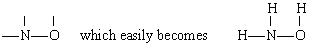# 6.15.2: Deciding on a Skeleton Structure

$$\newcommand{\vecs}{\overset { \rightharpoonup} {\mathbf{#1}} }$$ $$\newcommand{\vecd}{\overset{-\!-\!\rightharpoonup}{\vphantom{a}\smash {#1}}}$$$$\newcommand{\id}{\mathrm{id}}$$ $$\newcommand{\Span}{\mathrm{span}}$$ $$\newcommand{\kernel}{\mathrm{null}\,}$$ $$\newcommand{\range}{\mathrm{range}\,}$$ $$\newcommand{\RealPart}{\mathrm{Re}}$$ $$\newcommand{\ImaginaryPart}{\mathrm{Im}}$$ $$\newcommand{\Argument}{\mathrm{Arg}}$$ $$\newcommand{\norm}{\| #1 \|}$$ $$\newcommand{\inner}{\langle #1, #2 \rangle}$$ $$\newcommand{\Span}{\mathrm{span}}$$ $$\newcommand{\id}{\mathrm{id}}$$ $$\newcommand{\Span}{\mathrm{span}}$$ $$\newcommand{\kernel}{\mathrm{null}\,}$$ $$\newcommand{\range}{\mathrm{range}\,}$$ $$\newcommand{\RealPart}{\mathrm{Re}}$$ $$\newcommand{\ImaginaryPart}{\mathrm{Im}}$$ $$\newcommand{\Argument}{\mathrm{Arg}}$$ $$\newcommand{\norm}{\| #1 \|}$$ $$\newcommand{\inner}{\langle #1, #2 \rangle}$$ $$\newcommand{\Span}{\mathrm{span}}$$$$\newcommand{\AA}{\unicode[.8,0]{x212B}}$$

Back to Writing Lewis Structures for Molecules

The skeleton structure of a covalent molecule can often be determined by considering the valences of the constituent atoms. Usually the atom which forms the largest number of bonds is found in the center of the skeleton, where it can connect to the maximum number of other atoms.

##### structural formula.

Solution There are several possible ways to link the atoms togetherDiagram 1 shows a central O atom bonded to H and C l. Second diagram shows a central C l atom bonded to H and O. Diagram 3 shows a central H atom bonded to C l and O. Fourth diagram shows H, O, and C l bonded in triangular cyclic structure.

The usual valence of H is 1, and so structures 3 and 4, which have two bonds to H, may be eliminated. The usual valence of Cl is also 1, and so structure 2 may also be ruled out. Structure 1 shows H forming one bond, Cl forming one, and O forming two, in agreement with the usual valences, and so it is chosen.

The total number of valence electrons available is 1 from H plus 6 from O plus 7 from Cl, or 14. Filling these into the skeleton we haveA central O atom with two unpaired electrons on the top and bottom respectively. On the left, it shares a pair of electrons with H. On the right, it shares a pair of electrons with C l which has six other electrons in its outer shell.

Note that O, which had the largest valence, is in the center of the skeleton.

##### Example 2:

Draw a structural formula for hydroxylamine, NH3O.

Solution In this case N has the largest valence (3), followed by O (2) and H (1). Both N and O can form “bridges” between other atoms, but H cannot. Therefore we place N and O in the center of the skeleton to giveThe first diagram shows a central N bonded to O. The N atom has two other bonds available to be shared and the O has one electron. For this reason the central N and O can easily form three bonds with three hydrogen. Two is bonded to N and one is bonded to O.

by addition of the three H atoms.

There are a total of 5 + 3 + 6 = 14 valence electrons from N, 3H’s and O. These can be placed as follows:A central N shares two electrons each with the two H. A pair is shared with oxygen. A pair of electrons from N are unpaired. O shares a pair with another H. Two pairs of electrons from O are unpaired.

Once the Lewis diagram has been determined, the molecular formula is often rewritten to remind us of what the structural formula is. For example, the molecular formula for hydroxylamine is usually written NH2OH instead of NH3O to remind us that two H’s are bonded to N and one to O. It is assumed that the person reading the formula will realize that N and O each have one valence electron left to share with each other, connecting —NH2 with —OH. In some cases more than one skeleton structure will satisfy the valence of each atom and the octet rule as well. For example, you can verify that the molecular formula C2H6O corresponds to both of the following:Ethyl alcohol starts with a C bonded to three H. This is bonded to another C with two hydrogens bonded to it. This central C is also connected to O which is also bonded to H. Dimethyl ether has a central O bonded to a C H 3 group on each side.

In such a case we can only decide which molecular structure we have by experiment. The properties of ethyl alcohol when diluted with water and consumed are well known. Dimethyl ether is a gas. Like the diethyl ether used in operating rooms, it is highly explosive and can put you to sleep. Two molecules, such as dimethyl ether and ethyl alcohol, which have the same molecular formula but different structural formulas are said to be isomers.

Back to Writing Lewis Structures for Molecules

## Acknowledgements:

The C2H6O molecules and their skeletal structures were created using MolView.

This page titled 6.15.2: Deciding on a Skeleton Structure is shared under a CC BY-NC-SA 4.0 license and was authored, remixed, and/or curated by Ed Vitz, John W. Moore, Justin Shorb, Xavier Prat-Resina, Tim Wendorff, & Adam Hahn.